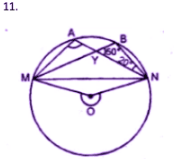Guru

# Question 5. (a) In the figure (i) given below, M, A, B, N are points on a circle having centre O. AN and MB cut at Y. If ∠NYB = 50° and ∠YNB = 20°, find ∠MAN and the reflex angle MON.

• 1

This is an important and exam oriented question from Chapter name- circles
Topic – Angle properties of circles
Chapter number- 15

In this question we have been given the figure of circle with certain information about the figure and we have to find the value of angle <MAN and <MON
ICSE Avichal publication
Understanding ICSE Mathematics
Question 5(a)

Share

1. Solution

(a) ∠NYB = 50°, ∠YNB = 20°.In
∆YNB,

NYB + ∠YNB + YBN = 180o

50+ 20+ ∠YBN = 180o

YBN + 70= 180o

YBN = 180– 70= 110o

But MAN = YBN

(Angles in the same segment)

MAN = 110o

Major arc MN subtend reflex MON at the

Centre and MAN at the remaining part of

the choice.

Reflex MAN at the remaining part of the circle

Reflex MON = 2 MAN = 2 × 110=220o

• 0